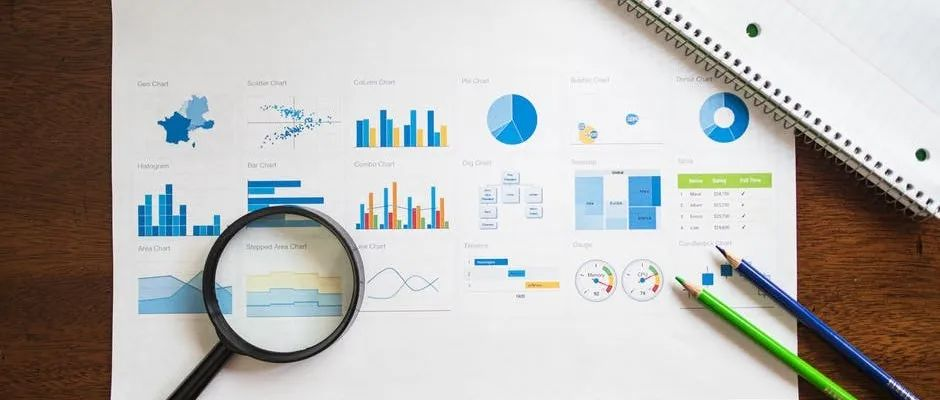## 探索Python强大引擎：将数据可视化提升到新高度的10个库

Power BI vs Tableau：数据可视化工具选哪个？

01 Plotly：前所未有的交互式可视化

``````import plotly.express as px
data = {'Category': ['A', 'B', 'C'], 'Value': [10, 15, 7]}
df = pd.DataFrame(data)
fig = px.bar(df, x='Category', y='Value')
fig.show()``````

02 Seaborn：美与简约的结合

``````import seaborn as sns
sns.boxplot(x='day', y='total_bill', data=tips)``````

03 Matplotlib：多功能可视化的经典选择

Matplotlib已经存在很长时间了，我相信它在数据分析师和可视化爱好者的心中占有特殊的位置。凭借其广泛的功能和灵活性，我认为Matplotlib允许你创建广泛的可视化。

``````import matplotlib.pyplot as plt
x = [1, 2, 3, 4, 5]
y = [10, 8, 6, 4, 2]
plt.plot(x, y)
plt.show()``````

04 Altair：快速声明式可视化

``````import altair as alt
from vega_datasets import data
cars = data.cars()
alt.Chart(cars).mark_circle().encode(
x='Horsepower',
y='Miles_per_Gallon',
color='Origin'
).interactive()``````

05 Bokeh：网络的交互式可视化

``````from bokeh.plotting import figure, show
from bokeh.models import HoverTool
from bokeh.sampledata.iris import flowers
p = figure(title='Iris Dataset', x_axis_label='Petal Length', y_axis_label='Petal Width')
p.circle(x='petal_length', y='petal_width', size=8, source=flowers)
show(p)``````

06 ggplot：利用R ggplot2的力量

``````from ggplot import *
df = pd.DataFrame({'x': [1, 2, 3, 4, 5], 'y': [10, 8, 6, 4, 2]})
ggplot(aes(x='x', y='y'), data=df) + geom_point()``````

07 NetworkX：可视化复杂网络

``````import networkx as nx
import matplotlib.pyplot as plt
G = nx.karate_club_graph()
nx.draw(G, with_labels=True)
plt.show()``````

08 Wordcloud：释放文本可视化的力量

``````from wordcloud import WordCloud
import matplotlib.pyplot as plt
text = "Lorem ipsum dolor sit amet, consectetur adipiscing elit."
wordcloud = WordCloud().generate(text)
plt.imshow(wordcloud, interpolation='bilinear')
plt.axis('off')
plt.show()``````

09 Pygal：交互式SVG图表

``````import pygal
bar_chart = pygal.Bar()
bar_chart.add('Series', [1, 3, 2, 5, 4])
bar_chart.render()``````

10 Folium：图表变得简单

``````import folium
m = folium.Map(location=[37.7749, -122.4194], zoom_start=12)